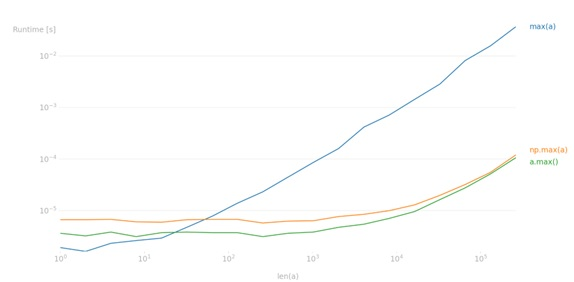# numpy.max() or max(), which one is faster?

Learn about the numpy.max() and max() functions, and learn which function is faster.
Submitted by Pranit Sharma, on March 01, 2023

NumPy is an abbreviated form of Numerical Python. It is used for different types of scientific operations in python. Numpy is a vast library in python which is used for almost every kind of scientific or mathematical operation. It is itself an array which is a collection of various methods and functions for processing the arrays.

## NumPy's max() or max(), which one is faster?

If we have a numpy array, we should use numpy.max() but if we have a built-in list then most of the time takes converting it into numpy.ndarray hence, we must use arr/list.max().

In terms of speed, both numpy.max() and arr.max() work similarly, however, max(arr) works much faster than these two methods.

To understand it with the help of visuals, we can use the python perfplot module to plot the time difference between these three.

## Python code to demonstrate numpy.max() or max(), which one is faster

```import numpy as np
import perfplot

b = perfplot.bench(
setup=np.random.rand,
kernels=[max, np.max, lambda arr: arr.max()],
labels=["max(a)", "np.max(a)", "a.max()"],
n_range=[2 ** k for k in range(25)],
xlabel="len(a)",
)
b.show()
```

Output:Languages: » C » C++ » C++ STL » Java » Data Structure » C#.Net » Android » Kotlin » SQL
Web Technologies: » PHP » Python » JavaScript » CSS » Ajax » Node.js » Web programming/HTML
Solved programs: » C » C++ » DS » Java » C#
Aptitude que. & ans.: » C » C++ » Java » DBMS
Interview que. & ans.: » C » Embedded C » Java » SEO » HR
CS Subjects: » CS Basics » O.S. » Networks » DBMS » Embedded Systems » Cloud Computing
» Machine learning » CS Organizations » Linux » DOS
More: » Articles » Puzzles » News/Updates